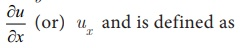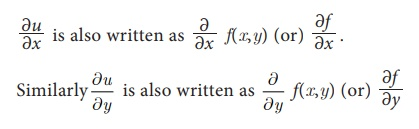Home | | Business Maths 11th std | Partial Derivatives

# Partial Derivatives

Partial derivative of a function of several variables is its derivative with respect to one of those variables, keeping other variables as constant.

Partial Derivatives

Partial derivative of a function of several variables is its derivative with respect to one of those variables, keeping other variables as constant. In this section, we will restrict our study to functions of two variables and their derivatives only.

Let u = f ( x , y)  be a function of two independent variables x and y.

The derivative of u with respect to x when x varies and y remains constant is called the partial derivative of u with respect to x, denoted byprovided the limit exists. Here Ōłåx is a small change in x

The derivative of u with respect to y, when y varies and x remains constant is called the partial derivative of u with respect to y, denoted byprovided the limit exists. Here Ōłåy is a small change in y.The process of finding a partial derivative is called partial differentiation.

Tags : Applications of Differentiation , 11th Business Mathematics and Statistics(EMS) : Chapter 6 : Applications of Differentiation
Study Material, Lecturing Notes, Assignment, Reference, Wiki description explanation, brief detail
11th Business Mathematics and Statistics(EMS) : Chapter 6 : Applications of Differentiation : Partial Derivatives | Applications of Differentiation